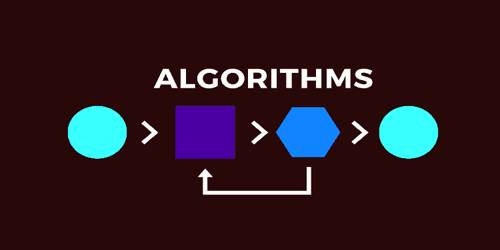# Differences between Algorithm and Flowchart

Differences between Algorithm and Flowchart

An algorithm is a well-defined list of steps for solving a particular problem. One major purpose of this text is to develop an efficient algorithm for the processing of our data. The time and space it uses are two major measures of the efficiency of an algorithm.

A flowchart is a diagram that depicts a process, system or computer algorithm. The flow chart symbols are linked together with arrows showing the process flow direction.

The differences between algorithm and flowchart are given below:

Algorithm

• Nature: Algorithm is a stepwise analysis of the work to be done.
• Representation: An algorithm is a precise rule specifying how to solve some problem.
• Steps: It is a well-defined list of steps for solving a particular problem.
• First: An algorithm is first represented in the form of a flowchart.
• Uses: Algorithm does not use a picture for representation.
• Sequence: It solves the problem by specified sequence.

Flowchart

• Nature: Flowchart is a pictorial representation of an algorithm. As flowchart is a picture of work to be done, it may be printed in our mind when we observed it.
• Representation: A flowchart is a diagram of the sequence of operations in a computer program or an accounting system.
• Steps: Graphical representation of algorithm is known as a flowchart.
• First: The flowchart is expressed in a programming language to prepare a computer program.
• Uses: Flowchart uses a diagram.
• Sequence: It solves the problem step by step using symbols. Flowchart uses a picture for representation.